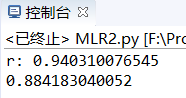# ML之MLiR：输入两个向量，得出两个向量之间的相关度

+关注继续查看

## 输出结果## 实现代码

import numpy as np

from astropy.units import Ybarn

import math

from statsmodels.graphics.tukeyplot import results

def computeCorrelation(X, Y):

xBar = np.mean(X)

yBar = np.mean(Y)

SSR = 0

varX = 0

varY = 0

for i in range(0 , len(X)):

diffXXBar = X[i] - xBar

diffYYBar = Y[i] - yBar

SSR += (diffXXBar * diffYYBar)

varX +=  diffXXBar**2

varY += diffYYBar**2

SST = math.sqrt(varX * varY)

return SSR / SST

testX = [1, 3, 8, 7, 9]

testY = [10, 12, 24, 21, 34]

print ("r:",computeCorrelation(testX, testY))

def polyfit(x,y,degree):

results={}

coeffs =np.polyfit(x,y,degree)

results['polynomial'] = coeffs.tolist()

p=np.poly1d(coeffs)

yhat=p(x)

ybar=np.sum(y)/len(y)

ssreg=np.sum((yhat-ybar)**2)

sstot=np.sum((y-ybar)**2)

results['determination']=ssreg/sstot

return results

print (polyfit(testX, testY, 1)["determination"])864 0Java笔记5：解决MyEclipse控制台输入中文乱码输出问题（Eclipse也可以用）
1047 03950 0Eclipse输入任意字母或指定字符出现提示框
916 01002 0Java中IO流，输入输出流概述与总结

1189 0Linux&shell之处理用户输入
823 09.2.1、Libgdx的输入处理之轮询
（官网：www.libgdx.cn） 轮询是检测输入设备的当前状态，比如特定的按键按下，屏幕第一个手指的位置等等。这是一个快速简单的处理用户输入的方式，并且应用到很多的游戏中。
779 01795 02901 0

1701

0

《SaaS模式云原生数据仓库应用场景实践》

《看见新力量：二》电子书